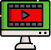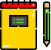VideosNotes

### Co-ordinate Geometry - 1

Co-ordinate Geometry
Coordinate geometry (or analytic geometry) is defined as the study of geometry using the coordinate points. Using coordinate geometry, it is possible to find the distance between two points, dividing lines in m:n ratio, finding the mid-point of a line, calculating the area of a triangle in the Cartesian plane, etc.
Views: N/A
Video Duration: 11:32 (Part 1 of 3)